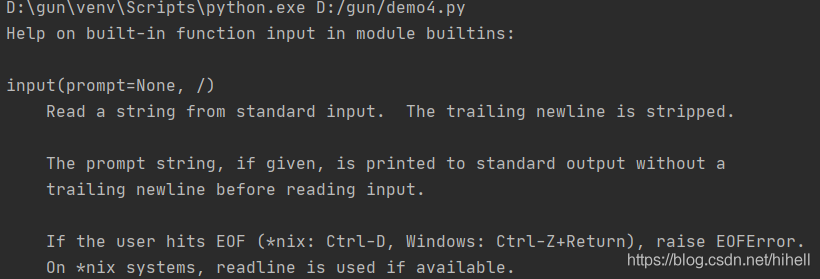2. 无门槛学会数据类型与输入、输出函数，滚雪球学 Python梦想橡皮擦94 0 0

# 二、Python 基本数据类型与输入、输出函数

## 2.1 基本数据类型

1. 数值数据类型，常见的是整数和浮点数
2. 布尔值数据类型，就是常说的真和假
3. 字符串数据类型

x = 10
print(type(x))

<class 'int'>

x = 10.1
print(type(x))


<class 'float'>

### 2.1.1 数值数据类型

x = 10.1
y = 10
z = x + y
print(type(z))

### 2.1.2 整数的不同形式表示

x = 10
print(bin(x))

0b1010

### 2.1.3 数据类型强制转换

x = 10.5
print(int(x))

int 其实也是 Python 的一个内置函数，它会尝试将任何数据类型的变量转换成整数，注意是任何数据类型的变量。

### 2.1.4 扩展几个数值数据类型常用的函数

• abs() 计算绝对值
• pow() 次方运算
• round() 四舍五入
• max() 取最大值
• min() 去最小值

# abs() 计算绝对值
a = -1
print(abs(a))
# pow() 次方运算
x = 2
c = 3
print(pow(x,c))
# round() 四舍五入

d = 34.6
print(round(d))
# max()  取最大值
print(max(1,2,3))
# min() 去最小值
print(min(9,10,6))


### 2.1.5 布尔值数据类型

x = True
print(int(x))
print(int(False))

### 2.1.6 字符串数据类型

my_name = "梦想橡皮擦"
print(my_name)

my_name = '梦想橡皮擦'
print(my_name)

my_name = '梦想"橡皮擦'
print(my_name)
print(type(my_name))

a = "my"
b = "name"

c = a+b
print(c)

a = 123
b = "name"
# str(a) 将整数变量a转换成字符串
c = str(a) + b
print(c)


my_str = """

"""
print(my_str)

a = 'I\'m a girl '
print(a)


a = r"I\nm a girl"
print(a)

I\nm a girl

print("#"*100)

## 2.2 输入与输出

help(print)

print(value, ..., sep=' ', end='\n', file=sys.stdout, flush=False)

• value 表示要输出的数据，可以多个，用逗号 , 分隔
• sep 输出多个数据时，分隔符号，默认是空格
• end 输出一行末尾输出的符号
• file 输出位置，默认输出控制台，就是黑窗口中，可以设置到具体文件
• flush 是否清除数据流缓冲区，默认为 False（该内容先不做涉及）

# 输出多个数据
print("hello","dream")

# 多个数据输出的分隔符
print("i","love",sep="#")

### 2.2.1 格式化 print 输出

• %d 整数输出
• %f 浮点数输出
• %x 十六进制输出
• %o 八进制
• %s 字符串输出，其实会这一个就行了

name = "橡皮擦"
age = 18
score = 100

# 格式化一个变量输出
print("我是 %s" % name)
# 格式化多个变量输出
print("我是 %s 今年 %d岁了，我考试得了%d分" % (name,age,score))


name = "橡皮擦"
age = 18
score = 100

print("我是 %s" % name)
print("我是 %s 今年 %d岁了，我考试得了%d分" % (name,"nnn",score))


name = "橡皮擦"
age = 18
score = 100

print("我是 %s" % name)
print("我是 %s 今年 %s岁了，我考试得了%d分" % (name,18,score))

### 2.2.2 format 函数

print("我是{}，今年{}，考试得了{}".format(name,age,score))

### 2.2.3 input 数据输入

input 执行的和 print 恰好想法，通过 help 查看一下：参数比较简单只有一个输入提示语。测试代码：

name = input("请输入你的姓名：")
print("输入的姓名为{}".format(name))

input 比较简单，本文先介绍这些。

## 2.3 这篇博客的总结

好好学下去 每天都有新打击O(∩_∩)O 哈哈~